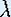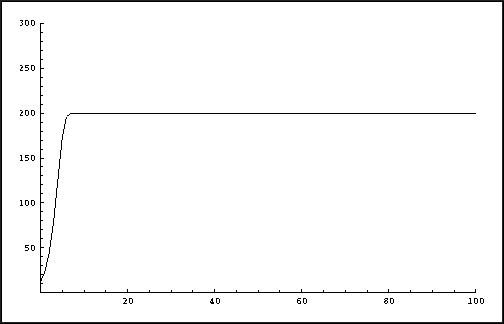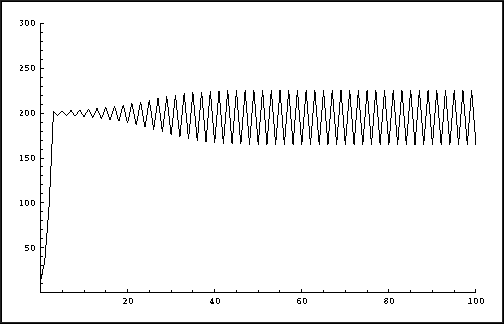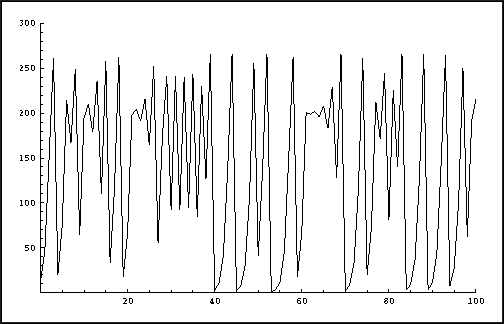Up: Logistic Growth Model

# One-Dimensional Dynamical Systems

## Part 2: Logistic Growth Model

#### Visualizing an orbit

Let us consider the evolution for p0 = 12, under the assumption that the university campus provides essential needs for maximally E = 200 squirrels. We set= 2, which corresponds to a growth rate of 1 baby per squirrel each year. What do you expect to happen as the years go by? We can visualize the orbit of p0 = 12 by plotting the iterates pn against the years n. In the following picture the evolution is shown over 100 years.Logistic growth with growth factor= 2.

The picture shows a monotonic increase towards the saturation value E. It is suprising to see what happens if we vary, but still use E = 200 and p0 = 12. The following two pictures show the results over 100 years for= 3.1 and= 4. In the former case the population eventually oscillates between two different values. The latter case shows an unexpected, complicated behavior: chaos!Logistic growth with growth factor= 3.1.Logistic growth with growth factor= 4.

Up: Logistic Growth ModelThe Geometry Center Home Page

Written by Hinke Osinga